# Tag: permutation representation

Here a collection of pdf-files of NeverEndingBooks-posts on groups, in reverse chronological order.

After a lengthy spring-break, let us continue with our course on noncommutative geometry and $SL_2(\mathbb{Z})$-representations. Last time, we have explained Grothendiecks mantra that all algebraic curves defined over number fields are contained in the profinite compactification
$\widehat{SL_2(\mathbb{Z})} = \underset{\leftarrow}{lim}~SL_2(\mathbb{Z})/N$ of the modular group $SL_2(\mathbb{Z})$ and in the knowledge of a certain subgroup G of its group of outer automorphisms
. In particular we have seen that many curves defined over the algebraic numbers $\overline{\mathbb{Q}}$ correspond to permutation representations of $SL_2(\mathbb{Z})$. The profinite compactification $\widehat{SL_2}=\widehat{SL_2(\mathbb{Z})}$ is a continuous group, so it makes sense to consider its continuous n-dimensional representations $\mathbf{rep}_n^c~\widehat{SL_2}$ Such representations are known to have a finite image in $GL_n(\mathbb{C})$ and therefore we get an embedding $\mathbf{rep}_n^c~\widehat{SL_2} \hookrightarrow \mathbf{rep}_n^{ss}~SL_n(\mathbb{C})$ into all n-dimensional (semi-simple) representations of $SL_2(\mathbb{Z})$. We consider such semi-simple points as classical objects as they are determined by – curves defined over $\overline{Q}$ – representations of (sporadic) finite groups – modlart data of fusion rings in RCTF – etc… To get a feel for the distinction between these continuous representations of the cofinite completion and all representations, consider the case of $\hat{\mathbb{Z}} = \underset{\leftarrow}{lim}~\mathbb{Z}/n \mathbb{Z}$. Its one-dimensional continuous representations are determined by roots of unity, whereas all one-dimensional (necessarily simple) representations of $\mathbb{Z}=C_{\infty}$ are determined by all elements of $\mathbb{C}$. Hence, the image of $\mathbf{rep}_1^c~\hat{\mathbb{Z}} \hookrightarrow \mathbf{rep}_1~C_{\infty}$ is contained in the unit circle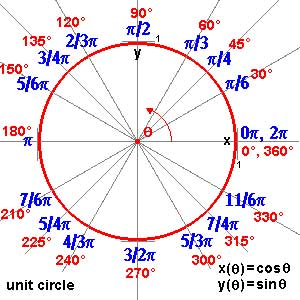and though these points are very special there are enough of them (technically, they form a Zariski dense subset of all representations). Our aim will be twofold : (1) when viewing a classical object as a representation of $SL_2(\mathbb{Z})$ we can define its modular content (which will be the noncommutative tangent space in this classical point to the noncommutative manifold of $SL_2(\mathbb{Z})$). In this way we will associate noncommutative gadgets to our classical object (such as orders in central simple algebras, infinite dimensional Lie algebras, noncommutative potentials etc. etc.) which give us new tools to study these objects. (2) conversely, as we control the tangentspaces in these special points, they will allow us to determine other $SL_2(\mathbb{Z})$-representations and as we vary over all classical objects, we hope to get ALL finite dimensional modular representations. I agree this may all sound rather vague, so let me give one example we will work out in full detail later on. Remember that one can reconstruct the sporadic simple Mathieu group $M_{24}$ from the dessin d’enfant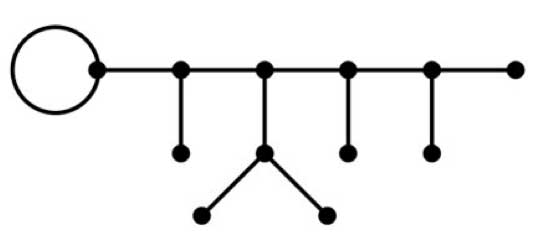This
dessin determines a 24-dimensional permutation representation (of
$M_{24}$ as well of $SL_2(\mathbb{Z})$) which
decomposes as the direct sum of the trivial representation and a simple
23-dimensional representation. We will see that the noncommutative
tangent space in a semi-simple representation of
$SL_2(\mathbb{Z})$ is determined by a quiver (that is, an
oriented graph) on as many vertices as there are non-isomorphic simple
components. In this special case we get the quiver on two points
$\xymatrix{\vtx{} \ar@/^2ex/[rr] & & \vtx{} \ar@/^2ex/[ll] \ar@{=>}@(ur,dr)^{96} }$ with just one arrow in each direction
between the vertices and 96 loops in the second vertex. To the
experienced tangent space-reader this picture (and in particular that
there is a unique cycle between the two vertices) tells the remarkable
fact that there is **a distinguished one-parameter family of
24-dimensional simple modular representations degenerating to the
permutation representation of the largest Mathieu-group**. Phrased
differently, there is a specific noncommutative modular Riemann surface
associated to $M_{24}$, which is a new object (at least as far
as I’m aware) associated to this most remarkable of sporadic groups.
Conversely, from the matrix-representation of the 24-dimensional
permutation representation of $M_{24}$ we obtain representants
of all of this one-parameter family of simple
$SL_2(\mathbb{Z})$-representations to which we can then perform
noncommutative flow-tricks to get a Zariski dense set of all
24-dimensional simples lying in the same component. (Btw. there are
also such noncommutative Riemann surfaces associated to the other
is what we will be doing in the upcoming posts (10) : explain what a
noncommutative tangent space is and what it has to do with quivers (11)
what is the noncommutative manifold of $SL_2(\mathbb{Z})$? and what is its connection with the Kontsevich-Soibelman coalgebra? (12)
is there a noncommutative compactification of $SL_2(\mathbb{Z})$? (and other arithmetical groups) (13) : how does one calculate the noncommutative curves associated to the Mathieu groups? (14) : whatever comes next… (if anything).

Last time we saw
that a curve defined over $\overline{\mathbb{Q}}$ gives rise
to a permutation representation of $PSL_2(\mathbb{Z})$ or one
of its subgroups $\Gamma_0(2)$ (of index 2) or
$\Gamma(2)$ (of index 6). As the corresponding
monodromy group is finite, this representation factors through a normal
subgroup of finite index, so it makes sense to look at the profinite
completion
of $SL_2(\mathbb{Z})$, which is the inverse limit
of finite
groups $\underset{\leftarrow}{lim}~SL_2(\mathbb{Z})/N$
where N ranges over all normalsubgroups of finite index. These
profinte completions are horrible beasts even for easy groups such as
$\mathbb{Z}$. Its profinite completion
is

$\underset{\leftarrow}{lim}~\mathbb{Z}/n\mathbb{Z} = \prod_p \hat{\mathbb{Z}}_p$

where the right hand side
product of p-adic integers ranges over all prime numbers! The
_absolute Galois group_
$G=Gal(\overline{\mathbb{Q}}/\mathbb{Q})$ acts on all curves
defined over $\overline{\mathbb{Q}}$ and hence (via the Belyi
maps ans the corresponding monodromy permutation representation) there
is an action of $G$ on the profinite completions of the
carthographic groups.

This is what Grothendieck calls anabelian
algebraic geometry

Returning to the general
case, since finite maps can be interpreted as coverings over
$\overline{\mathbb{Q}}$ of an algebraic curve defined over
the prime field $~\mathbb{Q}$ itself, it follows that the
Galois group $G$ of $\overline{\mathbb{Q}}$ over
$~\mathbb{Q}$ acts on the category of these maps in a
natural way.
For instance, the operation of an automorphism
$~\gamma \in G$ on a spherical map given by the rational
function above is obtained by applying $~\gamma$ to the
coefficients of the polynomials P , Q. Here, then, is that
mysterious group $G$ intervening as a transforming agent on
topologico- combinatorial forms of the most elementary possible
nature, leading us to ask questions like: are such and such oriented
maps ‚conjugate or: exactly which are the conjugates of a given
oriented map? (Visibly, there is only a finite number of these).
I considered some concrete cases (for coverings of low degree) by
various methods, J. Malgoire considered some others ‚ I doubt that
there is a uniform method for solving the problem by computer. My
reflection quickly took a more conceptual path, attempting to
apprehend the nature of this action of G.
One sees immediately
that roughly speaking, this action is expressed by a certain
outer action of G on the profinite com- pactification of the
oriented cartographic group $C_+^2 = \Gamma_0(2)$ , and this
action in its turn is deduced by passage to the quotient of the
canonical outer action of G on the profinite fundamental group
$\hat{\pi}_{0,3}$ of
$(U_{0,3})_{\overline{\mathbb{Q}}}$ where
$U_{0,3}$ denotes the typical curve of genus 0 over the
prime field Q, with three points re- moved.
This is how my
attention was drawn to what I have since termed anabelian
algebraic geometry
, whose starting point was exactly a study
(limited for the moment to characteristic zero) of the action of
absolute Galois groups (particularly the groups Gal(K/K),
where K is an extension of finite type of the prime field) on
(profinite) geometric fundamental groups of algebraic varieties
(defined over K), and more particularly (break- ing with a
well-established tradition) fundamental groups which are very far
from abelian groups (and which for this reason I call
anabelian).
Among these groups, and very close to
the group $\hat{\pi}_{0,3}$ , there is the profinite
compactification of the modular group $Sl_2(\mathbb{Z})$,
whose quotient by its centre ±1 contains the former as congruence
subgroup mod 2, and can also be interpreted as an oriented
cartographic group, namely the one classifying triangulated
oriented maps (i.e. those whose faces are all triangles or
monogons).

and a bit further, on page
250

I would like to conclude this rapid outline
with a few words of commentary on the truly unimaginable richness
of a typical anabelian group such as $SL_2(\mathbb{Z})$
doubtless the most remarkable discrete infinite group ever
encountered, which appears in a multiplicity of avatars (of which
certain have been briefly touched on in the present report), and which
from the point of view of Galois-Teichmuller theory can be
considered as the fundamental ‚building block‚ of the
Teichmuller tower
The element of the structure of
$Sl_2(\mathbb{Z})$ which fascinates me above all is of course
the outer action of G on its profinite compactification. By
Bielyi’s theorem, taking the profinite compactifications of subgroups
of finite index of $Sl_2(\mathbb{Z})$, and the induced
outer action (up to also passing to an open subgroup of G), we
essentially find the fundamental groups of all algebraic curves (not
necessarily compact) defined over number fields K, and the outer
action of $Gal(\overline{K}/K)$ on them at least it is
true that every such fundamental group appears as a quotient of one
of the first groups.
Taking the anabelian yoga
(which remains conjectural) into account, which says that an anabelian
algebraic curve over a number field K (finite extension of Q) is
known up to isomorphism when we know its mixed fundamental group (or
what comes to the same thing, the outer action of
$Gal(\overline{K}/K)$ on its profinite geometric
fundamental group), we can thus say that
all algebraic
curves defined over number fields are contained in the profinite
compactification $\widehat{SL_2(\mathbb{Z})}$ and in the
knowledge of a certain subgroup G of its group of outer
automorphisms!

To study the absolute
Galois group $Gal(\overline{\mathbb{\mathbb{Q}}}/\mathbb{Q})$ one
investigates its action on dessins denfants. Each dessin will be part of
a finite family of dessins which form one orbit under the Galois action
and one needs to find invarians to see whether two dessins might belong
to the same orbit. Such invariants are called _Galois invariants_ and
quite a few of them are known.

Among these the easiest to compute
are

• the valency list of a dessin : that is the valencies of all
vertices of the same type in a dessin
• the monodromy group of a dessin : the subgroup of the symmetric group $S_d$ where d is
the number of edges in the dessin generated by the partitions $\tau_0$
and $\tau_1$ For example, we have seen
before
that the two
Mathieu-dessins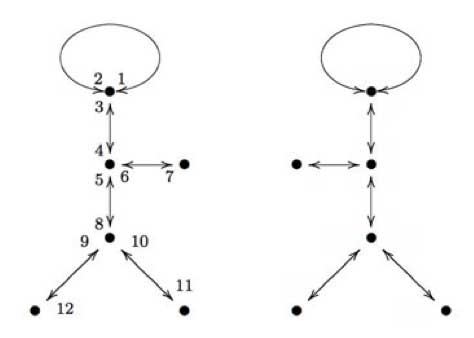form a Galois orbit. As graphs (remeber we have to devide each
of the edges into two and the midpoints of these halfedges form one type
of vertex, the other type are the black vertices in the graphs) these
are isomorphic, but NOT as dessins as we have to take the embedding of
them on the curve into account. However, for both dessins the valency
lists are (white) : (2,2,2,2,2,2) and (black) :
(3,3,3,1,1,1) and one verifies that both monodromy groups are
isomorphic to the Mathieu simple group $M_{12}$ though they are
not conjugated as subgroups of $S_{12}$.

Recently, new
Galois invariants were obtained from physics. In Children’s drawings
from Seiberg-Witten curves

the authors argue that there is a close connection between Grothendiecks
programme of classifying dessins into Galois orbits and the physics
problem of classifying phases of N=1 gauge theories…

Apart
from curves defined over $\overline{\mathbb{Q}}$ there are
other sources of semi-simple $SL_2(\mathbb{Z})$
representations. We will just mention two of them and may return to them
in more detail later in the course.

their representations
There are 26 exceptional finite simple groups
and as all of them are generated by two elements, there are epimorphisms
$\Gamma(2) \rightarrow S$ and hence all their representations
are also semi-simple $\Gamma(2)$-representations. In fact,
looking at the list of ‘standard generators’ of the sporadic
simples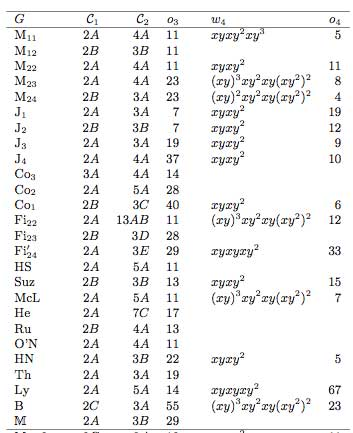(here the conjugacy classes of the generators follow the
notation of the Atlas project) we see that all but
possibly one are epimorphic images of $\Gamma_0(2) = C_2 \ast C_{\infty}$ and that at least 12 of then are epimorphic images
of $PSL_2(\mathbb{Z}) = C_2 \ast C_3$.

Rational conformal field theories Another
source of $SL_2(\mathbb{Z})$ representations is given by the
modular data associated to rational conformal field theories.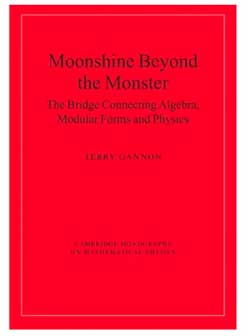These
representations also factor through a quotient by a finite index normal
subgroup and are therefore again semi-simple
$SL_2(\mathbb{Z})$-representations. For a readable
introduction to all of this see chapter 6 \”Modular group
representations throughout the realm\” of the
book Moonshine beyond the monster the bridge connecting algebra, modular forms and physics by Terry
Gannon
. In fact, the whole book
is a good read. It introduces a completely new type of scientific text,
that of a neverending survey paper…

Today we will explain how curves defined over
$\overline{\mathbb{Q}}$ determine permutation representations
of the carthographic groups. We have seen that any smooth projective
curve $C$ (a Riemann surface) defined over the algebraic
closure $\overline{\mathbb{Q}}$ of the rationals, defines a
_Belyi map_ $\xymatrix{C \ar[rr]^{\pi} & & \mathbb{P}^1}$ which is only ramified over the three points
$\\{ 0,1,\infty \\}$. By this we mean that there are
exactly $d$ points of $C$ lying over any other point
of $\mathbb{P}^1$ (we call $d$ the degree of
$\pi$) and that the number of points over $~0,1~$ and
$~\infty$ is smaller than $~d$. To such a map we
associate a _dessin d\’enfant_, a drawing on $C$ linking the
pre-images of $~0$ and $~1$ with exactly $d$
edges (the preimages of the open unit-interval). Next, we look at
the preimages of $~0$ and associate a permutation
$\tau_0$ of $~d$ letters to it by cycling
counter-clockwise around these preimages and recording the edges we
meet. We repeat this procedure for the preimages of $~1$ and
get another permutation $~\tau_1$. That is, we obtain a
subgroup of the symmetric group $\langle \tau_0,\tau_1 \rangle \subset S_d$ which is called the monodromy
group
of the covering $\pi$.For example, the
dessin on the right is
associated to a degree $8$ map $\mathbb{P}^1 \rightarrow \mathbb{P}^1$ and if we let the black (resp. starred) vertices be
the preimages of $~0$ (respectively of $~1$), then the
corresponding partitions are $\tau_0 = (2,3)(1,4,5,6)$
and $\tau_1 = (1,2,3)(5,7,8)$ and the monodromy group
is the alternating group $A_8$ (use
GAP ).

But wait! The map is also
ramified in $\infty$ so why don\’t we record also a
permutation $\tau_{\infty}$ and are able to compute it from
the dessin? (Note that all three partitions are needed if we want to
reconstruct $C$ from the $~d$ sheets as they encode in
which order the sheets fit together around the preimages). Well,
the monodromy group of a $\mathbb{P}^1$ covering ramified only
in three points is an epimorphic image of the fundamental
group
of the sphere
minus three points $\pi_1(\mathbb{P}^1 – { 0,1,\infty })$ That is, the group of all loops beginning and
ending in a basepoint upto homotopy (that is, two such loops are the
same if they can be transformed into each other in a continuous way
while avoiding the three points).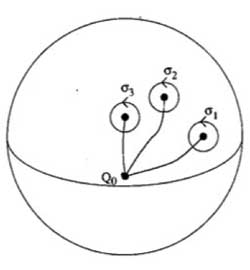This group is generated by loops
$\sigma_i$ running from the basepoint to nearby the i-th
point, doing a counter-clockwise walk around it and going back to be
basepoint $Q_0$ and the epimorphism to the monodromy group is given by sending

$\sigma_1 \mapsto \tau_0~\quad~\sigma_2 \mapsto \tau_1~\quad~\sigma_3 \mapsto \tau_{\infty}$

Now,
these three generators are not independent. In fact, this fundamental
group is

$\pi_1(\mathbb{P}^1 – \\{ 0,1,\infty \\}) = \langle \sigma_1,\sigma_2,\sigma_3~\mid~\sigma_1 \sigma_2 \sigma_3 = 1 \rangle$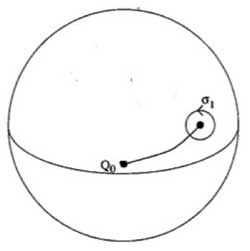To understand this, let us begin
with an easier case, that of the sphere minus one point. The fundamental group of the plane minus one point is
$~\mathbb{Z}$ as it encodes how many times we walk around the
point. However, on the sphere the situation is different as we can make
our walk around the point longer and longer until the whole walk is done
at the backside of the sphere and then we can just contract our walk to
the basepoint. So, there is just one type of walk on a sphere minus one
point (upto homotopy) whence this fundamental group is trivial. Next,
let us consider the sphere minus two points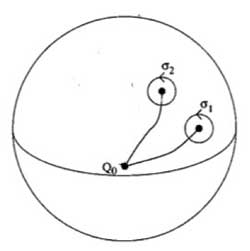Repeat the foregoing to the walk $\sigma_2$, that
is, strech the upper part of the circular tour all over the backside of
the sphere and then we see that we can move it to fit with the walk
$\sigma_1$ BUT for the orientation of the walk! That is, if we do this
modified walk $\sigma_1 \sigma_2^{\’}$ we just made the
trivial walk. So, this fundamental group is $\langle \sigma_1,\sigma_2~\mid~\sigma_1 \sigma_2 = 1 \rangle = \mathbb{Z}$ This is also the proof of the above claim. For,
we can modify the third walk $\sigma_3$ continuously so that
it becomes the walk $\sigma_1 \sigma_2$ but
with the reversed orientation ! As $\sigma_3 = (\sigma_1 \sigma_2)^{-1}$ this allows us to compute the
\’missing\’ permutation $\tau_{\infty} = (\tau_0 \tau_1)^{-1}$ In the example above, we obtain
$\tau_{\infty}= (1,2,6,5,8,7,4)(3)$ so it has two cycles
corresponding to the fact that the dessin has two regions (remember we
should draw ths on the sphere) : the head and the outer-region. Hence,
the pre-images of $\infty$ correspond to the different regions of the
dessin on the curve $C$. For another example,
consider the degree 168 map

$K \rightarrow \mathbb{P}^1$

which is the modified orbit map for the action of
$PSL_2(\mathbb{F}_7)$ on the Klein quartic.
The corresponding dessin is the heptagonal construction of the Klein
quartic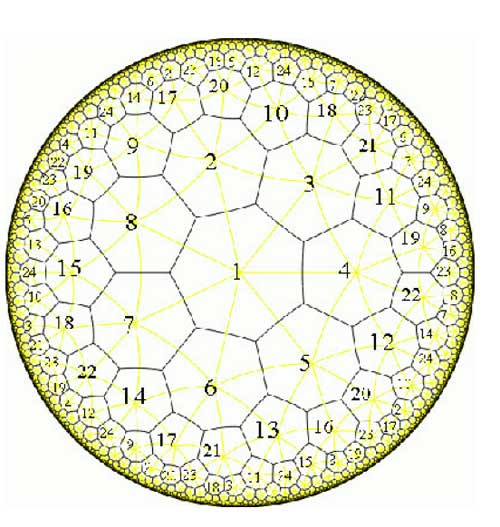Here, the pre-images of 1 correspond to the midpoints of the
84 edges of the polytope whereas the pre-images of 0 correspond to the
56 vertices. We can label the 168 half-edges by numbers such that
$\tau_0$ and $\tau_1$ are the standard generators b
resp. a of the 168-dimensional regular representation (see the atlas
page
).
Calculating with GAP the element $\tau_{\infty} = (\tau_0 \tau_1)^{-1} = (ba)^{-1}$ one finds that this permutation
consists of 24 cycles of length 7, so again, the pre-images of
$\infty$ lie one in each of the 24 heptagonal regions of the
Klein quartic. Now, we are in a position to relate curves defined
over $\overline{Q}$ via their Belyi-maps and corresponding
dessins to Grothendiecks carthographic groups $\Gamma(2)$,
$\Gamma_0(2)$ and $SL_2(\mathbb{Z})$. The
dessin gives a permutation representation of the monodromy group and
because the fundamental group of the sphere minus three
points $\pi_1(\mathbb{P}^1 – \\{ 0,1,\infty \\}) = \langle \sigma_1,\sigma_2,\sigma_3~\mid~\sigma_1 \sigma_2 \sigma_3 = 1 \rangle = \langle \sigma_1,\sigma_2 \rangle$ is the free group op two generators, we see that
any dessin determines a permutation representation of the congruence
subgroup $\Gamma(2)$ (see this
post
where we proved that this
group is free). A clean dessin is one for which one type of
vertex has all its valancies (the number of edges in the dessin meeting
the vertex) equal to one or two. (for example, the pre-images of 1 in
the Klein quartic-dessin or the pre-images of 1 in the monsieur Mathieu
example
) The corresponding
permutation $\tau_1$ then consists of 2-cycles and hence the
monodromy group gives a permutation representation of the free
product $C_{\infty} \ast C_2 = \Gamma_0(2)$ Finally, a clean dessin is said to be a
quilt dessin if also the other type of vertex has all its valancies
equal to one or three (as in the Klein quartic or Mathieu examples).
Then, the corresponding permutation has order 3 and for these
quilt-dessins the monodromy group gives a permutation representation of
the free product $C_2 \ast C_3 = PSL_2(\mathbb{Z})$ Next time we will see how this lead
Grothendieck to his anabelian geometric approach to the absolute Galois
group.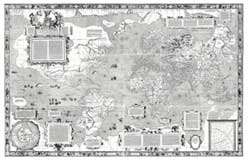Just as cartographers like
Mercator drew maps of
the then known world, we draw dessins
d ‘enfants
to depict the
associated algebraic curve defined over
$\overline{\mathbb{Q}}$.

In order to see that such a dessin
d’enfant determines a permutation representation of one of
Grothendieck’s cartographic groups, $SL_2(\mathbb{Z}), \Gamma_0(2)$ or $\Gamma(2)$ we need to have realizations of these
groups (as well as their close relatives
$PSL_2(\mathbb{Z}),GL_2(\mathbb{Z})$ and $PGL_2(\mathbb{Z})$) in
terms of generators and relations.

As this lesson will be rather
technical I’d better first explain what we will prove (so that you can
skip it if you feel comfortable with the statements) and why we want to
prove it. What we will prove in detail below is that these groups
can be written as free (or amalgamated) group products. We will explain
what this means and will establish that

$PSL_2(\mathbb{Z}) = C_2 \ast C_3, \Gamma_0(2) = C_2 \ast C_{\infty}, \Gamma(2) = C_{\infty} \ast C_{\infty}$

$SL_2(\mathbb{Z}) = C_4 \ast_{C_2} C_6, GL_2(\mathbb{Z}) = D_4 \ast_{D_2} D_6, PGL_2(\mathbb{Z}) = D_2 \ast_{C_2} D_3$

where $C_n$ resp.
$D_n$ are the cyclic (resp. dihedral) groups. The importance of these
facts it that they will allow us to view the set of (isomorphism classes
of) finite dimensional representations of these groups as
noncommutative manifolds . Looking at the statements above we
see that these arithmetical groups can be build up from the first
examples in any course on finite groups : cyclic and dihedral
groups.

Recall that the cyclic group of order n, $C_n$ is the group of
rotations of a regular n-gon (so is generated by a rotation r with
angle $\frac{2 \pi}{n}$ and has defining relation $r^n = 1$, where 1
is the identity). However, regular n-gons have more symmetries :
flipping over one of its n lines of symmetry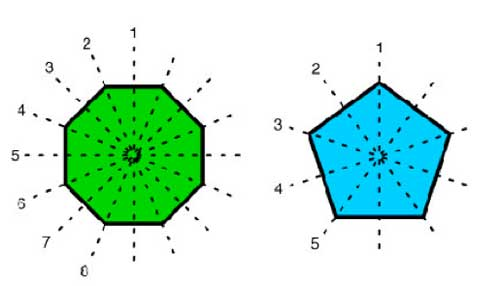The dihedral group $D_n$ is the group generated by the n
rotations and by these n flips. If, as before r is a generating
rotation and d is one of the flips, then it is easy to see that the
dihedral group is generated by r and d and satisfied the defining
relations

$r^n=1$ and $d^2 = 1 = (rd)^2$

Flipping twice
does nothing and to see the relation $~(rd)^2=1$ check that doing twice a
rotation followed by a flip brings all vertices back to their original
location. The dihedral group $D_n$ has 2n elements, the n-rotations
$r^i$ and the n flips $dr^i$.

In fact, to get at the cartographic
groups we will only need the groups $D_4, D_6$ and their
subgroups. Let us start by finding generators of the largest
group $GL_2(\mathbb{Z})$ which is the group of all invertible $2 \times 2$ matrices with integer coefficients.

Consider the
elements

$U = \begin{bmatrix} 0 & -1 \\ 1 & 0 \end{bmatrix}, V = \begin{bmatrix} 0 & 1 \\ -1 & 1 \end{bmatrix}/tex] and$R =
\begin{bmatrix} 0 & 1 \\ 1 & 0 \end{bmatrix} $and form the matrices$X = UV = \begin{bmatrix} 1 & -1 \\ 0 & 1
\end{bmatrix}, Y = VU = \begin{bmatrix} 1 & 0 \\ 1 & 1
\end{bmatrix} $By induction we prove the following relations in$GL_2(\mathbb{Z}) X^n \begin{bmatrix} a & b \\ c & d
\end{bmatrix} = \begin{bmatrix} a-nc & b-nd \\ c & d \end{bmatrix} $and$\begin{bmatrix} a & b \\ c& d \end{bmatrix} X^n =
\begin{bmatrix} a & b-na \\ c & d-nc \end{bmatrix} Y^n \begin{bmatrix} a & b \\ c & d \end{bmatrix} =
\begin{bmatrix} a & b \\ c+na & d+nb \end{bmatrix} $and$\begin{bmatrix} a & b \\ c & d \end{bmatrix} Y^n = \begin{bmatrix}
a+nb & b \\ c+nd & d \end{bmatrix} $The determinant ad-bc of a matrix in$GL_2(\mathbb{Z}) $must be$\pm 1 $whence all rows and columns of$\begin{bmatrix} a & b \\ c & d \end{bmatrix} \in
GL_2(\mathbb{Z}) $consist of coprime numbers and hence a and c can be reduced modulo each other by left multiplication by a power of X or Y until one of them is zero and the other is$\pm 1 $. We may even assume that$a = \pm 1 $(if not, left multiply with U). So, by left multiplication by powers of X and Y and U we can bring any element of$GL_2(\mathbb{Z}) $into the form$\begin{bmatrix}
\pm 1 & \beta \\ 0 & \pm 1 \end{bmatrix} $and again by left multiplication by a power of X we can bring it in one of the four forms$\begin{bmatrix} \pm 1 & 0 \\ 0 & \pm 1 \end{bmatrix}
= { 1,UR,RU,U^2 } $This proves that$GL_2(\mathbb{Z}) $is generated by the elements U,V and R. Similarly, the group$SL_2(\mathbb{Z}) $of all$2 \times 2 $integer matrices with determinant 1 is generated by the elements U and V as using the above method and the restriction on the determinant we will end up with one of the two matrices${ \begin{bmatrix} 1 & 0 \\ 0 & 1
\end{bmatrix},\begin{bmatrix} -1 & 0 \\ 0 & -1 \end{bmatrix} } =
{ 1,U^2 } $so we never need the matrix R. As for relations, there are some obvious relations among the matrices U,V and R, namely$U^2=V^3 $and$1=U^4=R^2=(RU)^2=(RV)^2

The
real problem is to prove that all remaining relations are consequences
of these basic ones. As R clearly has order two and its commutation
relations with U and V are just $RU=U^{-1}R$ and $RV=V^{-1}R$ we can
pull R in any relation to the far right and (possibly after
multiplying on the right with R) are left to prove that the only
relations among U and V are consequences of $U^2=V^3$ and
$U^4=1=V^6$.

Because $U^2=V^3$ this element is central in the
group generated by U and V (which we have seen to be
$SL_2(\mathbb{Z})$) and if we quotient it out we get the modular
group

$\Gamma = PSL_2(\mathbb{Z})$

Hence in order to prove our claim
it suffices that

$PSL_2(\mathbb{Z}) = \langle \overline{U},\overline{V} : \overline{U}^2=\overline{V}^3=1 \rangle$

Phrased differently, we have to show that
$PSL_2(\mathbb{Z})$ is the free group product of the cyclic groups of
order two and three (those generated by $u = \overline{U}$ and
$v=\overline{V}$) $C_2 \ast C_3$

Any element of this free group
product is of the form $~(u)v^{a_1}uv^{a_2}u \ldots uv^{a_k}(u)$ where beginning and trailing u are optional and
all $a_i$ are either 1 or 2.

So we have to show that in
$PSL_2(\mathbb{Z})$ no such word can give the identity
element. Today, we will first sketch the classical argument based
on the theory of groups acting on trees due to Jean-Pierre
Serre
and Hyman Bass. Tomorrow, we will give a short elegant proof due to
Roger Alperin and draw
consequences to the description of the carthographic groups as
amalgamated free products of cyclic and dihedral groups.

Recall
that $GL_2(\mathbb{Z})$ acts via Moebius
transformations
on
the complex plane $\mathbb{C} = \mathbb{R}^2$ (actually it is an
action on the Riemann sphere $\mathbb{P}^1_{\mathbb{C}}$) given by the
maps

$\begin{bmatrix} a & b \\ c & d \end{bmatrix}.z = \frac{az+b}{cz+d}$

Note that the action of the
center of $GL_2(\mathbb{Z})$ (that is of $\pm \begin{bmatrix} 1 & 0 \\ 0 & 1 \end{bmatrix}$) acts trivially, so it is really an action of
$PGL_2(\mathbb{Z})$.

As R interchanges the upper and lower half-plane
we might as well restrict to the action of $SL_2(\mathbb{Z})$ on the
upper-halfplane $\mathcal{H}$. It is quite easy to see that a
fundamental domain
for this action is given by the greyed-out area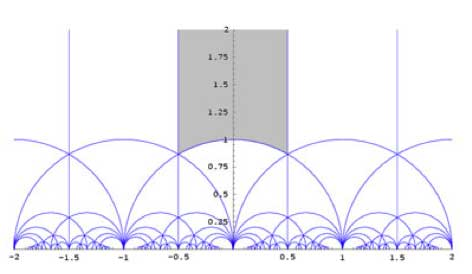To see that any $z \in \mathcal{H}$ can be taken into this
region by an element of $PSL_2(\mathbb{Z})$ note the following two
Moebius transformations

$\begin{bmatrix} 1 & 1 \\ 0 & 1 \end{bmatrix}.z = z+1$ and $\begin{bmatrix} 0 & 1 \\ -1 & 0 \end{bmatrix}.z = -\frac{1}{z}$

The first
operation takes any z into a strip of length one, for example that
with Re(z) between $-\frac{1}{2}$ and $\frac{1}{2}$ and the second
interchanges points within and outside the unit-circle, so combining the
two we get any z into the greyed-out region. Actually, we could have
taken any of the regions in the above tiling as our fundamental domain
as they are all translates of the greyed-out region by an element of
$PSL_2(\mathbb{Z})$.

Of course, points on the boundary of the
greyed-out fundamental region need to be identified (in order to get the
identification of $\overline{\mathcal{H}/PSL_2(\mathbb{Z})}$ with the
Riemann sphere $S^2=\mathbb{P}^1_{\mathbb{C}}$). For example, the two
halves of the boundary by the unit circle are interchanged by the action
of the map $z \rightarrow -\frac{1}{z}$ and if we take the translates under
$PSL_2(\mathbb{Z})$ of the indicated circle-part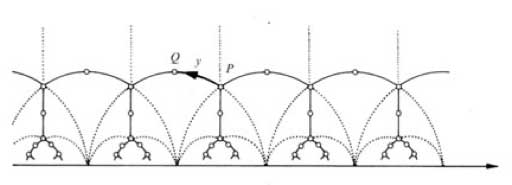we get a connected tree with fundamental domain the circle
part bounded by i and $\rho = \frac{1}{2}+\frac{\sqrt{3}}{2} i$.
Calculating the stabilizer subgroup of i (that is, the subgroup of
elements fixing i) we get that this subgroup
is $\langle u \rangle = C_2$ whereas the stabilizer subgroup of
$\rho$ is $\langle v \rangle = C_3$.

Using this facts and the general
results of Jean-Pierre Serres book Trees
one deduces that $PSL_2(\mathbb{Z}) = C_2 \ast C_3$
and hence that the obvious relations among U,V and R given above do
indeed generate all relations.